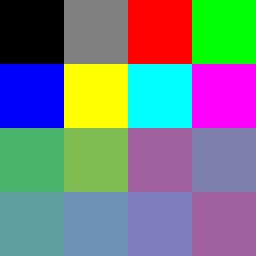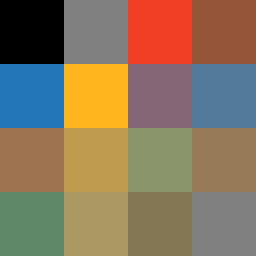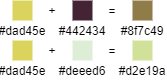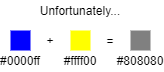## Processing 笔记本 (2)• 类和对象
• 如何显示对象
• 从文件读取

### 需求和交互设计（打草稿！）

• 正方形，划分成 16 格，每格里有颜色或空白
• 左键混色（落笔），右键吸色（蘸取）
• 颜料转移时会被稀释，便于控制添加颜色的量（后来发现很难做，要重构）

### 程序框架

import java.util.*;
import java.text.*;

// 记录当前颜色和透明度的全局变量
color brush_color = color(0, 0, 0);
float brush_alpha = 0.0;
// TODO  我们还需要定义一个 Paint 类来储存和显示颜料，过会儿再说
// Paint[] palette = new Paint ;

// 这是启动时执行的函数，用于初始化一些数据
void setup() {
// 分辨率 256*256，每格宽高 64
size(256, 256);
// TODO 在这里初始化和显示所有颜料
}

// 每帧更新画布的函数
void draw() {
// TODO 每帧画背景和颜料
}

// 每次鼠标点击后都会触发的函数
void mousePressed(){
if (mouseButton == LEFT) {
// 点击左键混色
}
else if (mouseButton == RIGHT) {
// 点击右键取色
}
}


int get_color_index(int x, int y) {
int row = y / 64;
int col = x / 64;
return row * 4 + col;
}


### Paint 类

• 当前颜色
• 当前透明度
• 所在的格子编号（用于绘制该对象）

• 混色（改变当前颜色）
• 吸色
public class Paint {
// 颜料对象的属性
color paint_color = color(0,0,0);
float paint_alpha = 0.0;
int index = 0;

// 构造方法，用于初始化
Paint(color c, float a, int i) {
// ...
}

void mix() {
// 混色
}

void pick() {
// 取色
}

// 用于在屏幕上绘制该颜色
void display() {
// ...
}
}


### 从文件加载色板

Processing 支持从 sketch 项目文件夹读取文本、图像等文件，所以我打算让它从外部批量读取色板，方便修改。

txt 文件格式如下，每行一个颜色，用空格分隔 rgb：

255 0 0
0 255 0
0 0 255


// 墨和水是两个基础颜料，且需要手动调整透明度，故 hardcode 之
palette = new Paint(color(0,0,0), 1.0, 0);
palette = new Paint(color(255,255,255), 0.0, 1);

// 从 palette.txt 加载，存在一个字符串 array 里
for (int i = 0; i < min(lines.length, 14); i++) {
int[] rgb = int(split(lines[i], ' '));
// 初始化颜色
palette[i+2] = new Paint(color(rgb, rgb, rgb), 1.0, i+2);
}

// 初始化剩下的空白格子
for (int i = lines.length; i < 14; i++){
palette[i+2] = new Paint(color(255,255,255), 0.0, i+2);
}


### 混色算法分析

#### Additive blending: the naive approach

void mix() {
paint_color = lerpColor(paint_color, brush_color, 0.5); // 暂且略过透明度计算
paint_alpha = (brush_alpha + paint_alpha) / 2;
display();
}（为什么蓝黄相加格外经典：因为在大部分人的生活经验里，蓝加黄就应该是绿色！相比之下红和天蓝、绿和粉红相加得到灰色，好像就没那么不可接受。）

#### Subtractive blending: CMYK

RGB 模型的乘法混合（正片叠底）解决了普通补色的混合，但依然无法解决蓝黄问题，它们相乘后会变成 #000000 也就是黑色。这并不是我们想看到的，所以否决。

CMYK 模型的蓝色 #00f 和黄色 #ff0 分别对应 (1, 1, 0, 0) 和 (0, 0, 1, 0)，CMYK 的加法运算等于 RGB 的乘法运算，故得到 (1, 1, 1, 0)，也是纯黑色。

The whole idea with RGB to CMYK conversion is to create a CMYK image which on print will look as similar as possible to the original RGB image.

A CMYK color is not an objective color, it's simply a set of technical instructions for the printing device on which halftone percentages to use.1. 先把所有的白色抠出来 $rgb_{RYB} –= min(rgb_{RGB})$
2. 从红色分量里把黄色抠出来 $r_{RYB} = r_{RGB} – min(r_{RGB}, g_{RGB})$，黄色晾干备用（因为 RGB 的黄色是红绿组成的）
3. 从绿色分量里把黄色抠出来，剩下的绿色晾干备用 $g_{RGB} – min(r_{RGB}, g_{RGB})$
4. 把这部分多余的绿色和蓝色搓在一起，形成新的蓝色分量 $b_{RYB} = (b_{RGB} + g_{RGB} – min(r_{RGB}, g_{RGB}) / 2$（因为 RGB 的绿色要拆进 RYB 的蓝和黄）
5. 把原本的绿色和之前抠出来的黄色搓在一起，形成新的黄色分量 $y_{RYB} = (g_{RGB} + min(r_{RGB}, g_{RGB}) / 2$
6. 最后，加回去一个黑色 $rgb_{RYB} += min(1 – rgb_{RGB})$

（🚧 施工中）

### Next

import java.util.*;
import java.text.*;

color brush_color = color(0, 0, 0);
float brush_alpha = 0.0;
Paint[] palette = new Paint ;

void setup() {
size(256, 256);

palette = new Paint(color(0,0,0), 1.0, 0);
palette = new Paint(color(255,255,255), 0.0, 1);

for (int i = 0; i < min(lines.length, 14); i++) {
int[] rgb = int(split(lines[i], ' '));
palette[i+2] = new Paint(color(rgb, rgb, rgb), 1.0, i+2);
}

for (int i = lines.length; i < 14; i++){
palette[i+2] = new Paint(color(255,255,255), 0.0, i+2);
}
}

void draw() {
background(128);
for (int i=0; i < palette.length; i++) {
palette[i].display();
}
}

void mousePressed(){
int idx = get_color_index(mouseX, mouseY);
if (mouseButton == LEFT) {
palette[idx].mix();
}
else if (mouseButton == RIGHT) {
palette[idx].pick();
}
}

int get_color_index(int x, int y) {
int row = y / 64;
int col = x / 64;
return row * 4 + col;
}

public class Paint {
color paint_color = color(0,0,0);
float paint_alpha = 0.0;
int index = 0;

Paint(color c, float a, int i) {
paint_color = c;
paint_alpha = a;
index = i;
}

void mix() {
float weight = constrain(brush_alpha / (paint_alpha + brush_alpha), 0.0, 1.0);
paint_color = lerpColor(paint_color, brush_color, weight);
paint_alpha = (weight + paint_alpha) / 2;
display();
}

void pick() {
brush_color = paint_color;
brush_alpha = paint_alpha;
}

void display() {
noStroke();
fill(paint_color, paint_alpha * 255);
rect(index % 4 * 64, index / 4 * 64, 64, 64);
}
}


color mixRYB(color c1, color c2, float w) {
color c1RYB = RGB2RYB(c1);
color c2RYB = RGB2RYB(c2);
color cRYB = lerpColor(c1RYB, c2RYB, w);
color c = RYB2RGB(cRYB);
return c;
}

// algorithm: http://nishitalab.org/user/UEI/publication/Sugita_SIG2015.pdf
// code: http://www.deathbysoftware.com/colors/index.html

color RGB2RYB(color rgb){
float r = red(rgb);
float g = green(rgb);
float b = blue(rgb);
float w = min(r,g,b);
r -= w;
g -= w;
b -= w;
float maxg = max(r,g,b);
float y = min(r,g);
r -= y;
g -= y;
if (b > 0 && g > 0) {
b /= 2;
g /= 2;
}
y += g;
b += g;
float maxy = max(r,y,b);
if (maxy > 0) {
float n = maxg / maxy;
r *= n;
y *= n;
b *= n;
}
color ryb = color(r+w, y+w, b+w);
return ryb;
}

color RYB2RGB(color ryb){
float r = red(ryb);
float y = green(ryb);
float b = blue(ryb);
float w = min(r,y,b);
r -= w;
y -= w;
b -= w;
float maxy = max(r,y,b);
float g = min(y,b);
y -= g;
b -= g;
if (b > 0 && g > 0) {
b *= 2;
g *= 2;
}
r += y;
g += y;
float maxg = max(r,g,b);
if (maxg > 0) {
float n = maxy / maxg;
r *= n;
g *= n;
b *= n;
}
color rgb = color(r+w, g+w, b+w);
return rgb;
}